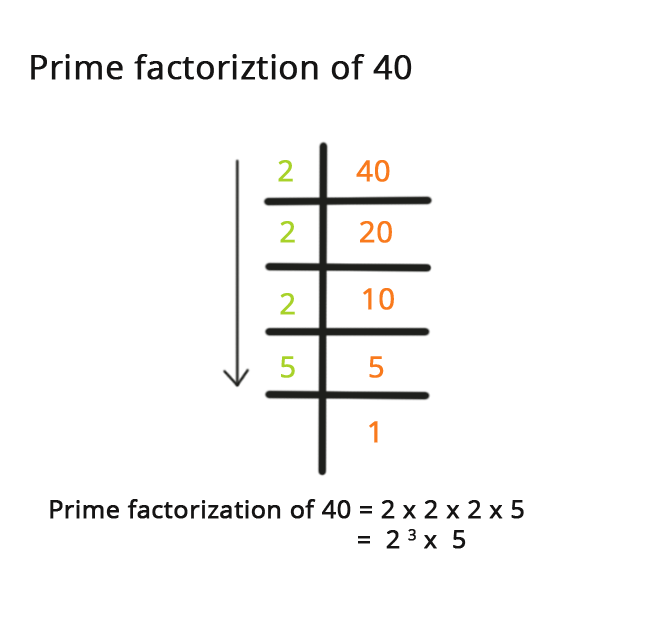• Call Now

1800-102-2727•

# Prime Factorization - Defintion, how does it work, Numbers Prime Factorization Table, Factor Tree and Division Method

A number can be expressed as a product of its prime components using prime factorization. A prime number is one with only two factors: one and the number itself. Let's look at the number 30 as an example. Although we know that 30 equals 5 + 6, 6 is not a prime number. Because 3 and 2 are prime integers, the number 6 is written as 2 3.

## What is Prime Factorization, and how does it work?

Prime factorization is the technique of expressing a number as the product of prime numbers. Prime numbers, such as 2, 3, 5, 7, 11, 13, 17, 19, and so on, contain just two factors: one and the number itself. The term "prime factorization" refers to the representation of a number as a product of prime numbers. Prime factorization of 40, for example, is the representation of 40 as a product of prime numbers, and it may be done as -

Numbers Prime Factorization
36 2 ×2×3×3
24 2×2×2× 3
60 2×2×3×5
18 2×3×3
72 2×2×2×3×3
45 3×3× 5
40 2×2×2×5
50 2×5×5
48 2×2×2×2×3
30 2×3×5
42 2×3×7## Factor Tree Method for Prime Factorization

The factor tree technique involves finding a number's factors and then factorizing those numbers until we reach prime numbers. Follow the steps below to determine a number's prime factorization using the factor tree method:
Step 1: Think of the number as the root of the tree at the very top of the factor tree.
Step 2: Then, as the tree's branches, put down the matching pair of components.
Step 3: Factorize the composite factors discovered in step 2 and write the pair of factors down as the tree's next branches.
Step 4: Repeat step 3 until all of the composite factors' prime factors are found.

## Prime Factorization's Division Method

By dividing a big number by prime numbers, the division technique may be used to discover the prime factors of a large number. To get a number's prime factors using the division technique, follow the steps below:
Step 1: Divide the number by the lowest prime number in such a way that the smallest prime number entirely divides the number.
Step 2: Subtract the lowest prime number from the quotient of step 1.
Step 3: Keep repeating steps 2 and 3 until the quotient equals one.
Step 4: Finally, multiply all of the divisors of the division by their prime factors.Talk to our expert
Resend OTP Timer =
By submitting up, I agree to receive all the Whatsapp communication on my registered number and Aakash terms and conditions and privacy policy